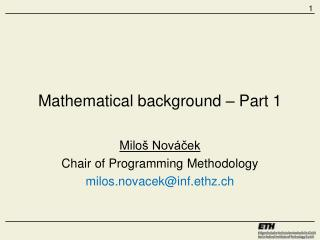# Mathematical background – Part 1 - PowerPoint PPT PresentationDownload PresentationMathematical background – Part 1

Mathematical background – Part 1Download Presentation## Mathematical background – Part 1

- - - - - - - - - - - - - - - - - - - - - - - - - - - E N D - - - - - - - - - - - - - - - - - - - - - - - - - - -
##### Presentation Transcript

1. Mathematical background – Part 1 Miloš Nováček Chair of Programming Methodology milos.novacek@inf.ethz.ch

2. Classes • Every Tuesday 1pm – 2pm (starting 1.15pm) • Hand in your solution to me via email milos.novacek@inf.ethz.ch by Monday 9am • Homework is optional but helpful • You can submit also partial solutions • Master solutions and slides from classes will be published after each class on the course web-page http://www.pm.inf.ethz.ch/education/courses/spa/exercises • Feedback!!!

3. Any questions about the content of the last lecture?

4. Exercise 1 Show that on is a partial order.

5. on is a partial order • As pointed out by Stephan at the lecturewe can use the above definition and use the fact that logical implication () is already partial order. Hence, we have a one step proof. • Definition of

6. Exercise 1 - proof • By definition of partial order, prove that • is reflexive • is antisymmetric • Statement: • Proof: By definition of, we have to prove that. • This is true since trivially implies that , so the right side of the implication is true. • Statement: • Proof: • Therefore, we have • Hence, .

7. Exercise 1 – proof (cont’d) • is transitive • Hence, on is a partial order. • Statement: • Proof: By definition of, we know that • Therefore, by transitive property of logical implication we know that • that, by definition of, means that

8. Exercise 2 Show that the inverse of a partial order on a set is a partial order on . Notation:

9. Inverse relation Definition: Let be a binary relation. Then is an inverse relation of the relation . Furthermore, .

10. Exercise 2 - proof • Let be a poset. Then we need to show that the inverse relation • is reflexive • is antisymmetric • Statement: • Proof: Since than so by the definition of an inverse relation • Statement: • Proof: • Let and . • Then and . • Hence, by antisymmetry of .

11. Exercise 2 – proof (cont’d) • is transitive • Hence, inverse of a partial order on a set is a partial order on . • Statement: • Proof: By definition of , we know that • Therefore, by transitivity of , we know that . • Hence, by the definition of the inverse of a relation.

12. Exercise 3 Show that is glb of .

13. Exercise 3 - proof • is the glb of iff: • (lower bound) • (greatest) • is glb of • Statement: • Proof: By definition of • If then , that is, . • By definition of , and . • Statement • Proof: • Combining:By def. of: • By def. of we have that Can also be proven by contradiction.

14. Questions?